Volume 1, Number 2, pp. 10-14, 1999.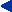Home  Current Issue  Table of Contents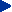De Peralta Menendez and S. L. Gonzalez Andino

Appendix I

Introduction

This Appendix extends the results presented in . Here, the following additional instantaneous, 3D, discrete, linear solutions for the EEG inverse problem are considered:

1. Weighted minimum norm, with weights that normalize (in the Lp - norm sense) the lead field.

2. LORETA without lead field normalization, with Laplacian having null current density beyond boundary.

3. LORETA without lead field normalization, with singular Laplacian having arbitrary current density beyond boundary.

1. Methods

The reader must refer to  for background and notation.

One type of generalized minimum norm inverse problem is: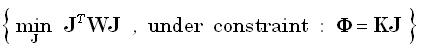(1)

for any given positive definite matrix W of dimension (3M)•(3M). The solution is: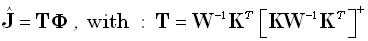(2)

where [KW-1KT]+ denotes the Moore-Penrose pseudoinverse of [KW-1KT].

1.1. Weighted minimum norm, with weights that normalize (in the Lp - norm sense) the lead field

In this case: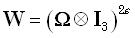(3)

where ε ≥ 0 is an exponent, ⊗ denotes the Kronecker product, I3 is the identity 3•3 -matrix, and Ω is a diagonal M•M -matrix with: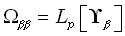(4)

which denotes the Lp norm, for any p > 0 , of the (3N)•1 vector defined by: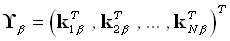(5)

1. The particular case ε = 0 corresponds to the classical "minimum norm".

2. The particular case ε = 1 , p = 2 corresponds to the classical "weighted minimum norm".

3. This version can be applied to any head geometry, i.e., it is not limited to spherical heads where concepts such as "radius" exist.

1.3. LORETA without lead field normalization, with Laplacian having null current density beyond boundary

In this case: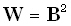(6)

where the matrix B implements a discrete spatial Laplacian operator. It should be emphasized that such a choice for B produces the smoothest possible inverse solution. This is because the inverse matrix, i.e. B-1 , implements a discrete spatial smoothing operator. For a solution space given by a regular cubic 3D grid, with minimum inter-grid-point distance "d", the Laplacian operator used in practice is defined as: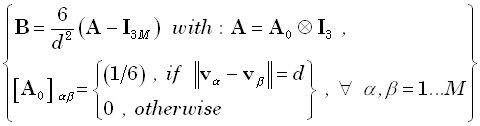(7)

Equation (7) corresponds exactly to the Laplacian operator implicitly defined in  (see Eq. (2) therein). The explicit definition of the Laplacian is included here (Equation (7)) for the benefit of readers that may be interested in implementing LORETA correctly.

An important comment:

This implements LORETA, without weights, and with boundary condition different from that used previously.

1.4. LORETA without lead field normalization, with singular Laplacian having arbitrary current density beyond boundary

In this case: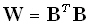(8)

where the matrix B implements the following discrete spatial Laplacian operator: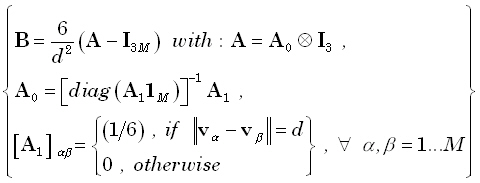(9)

Equation (9) corresponds exactly to the Laplacian operator implicitly defined and used in  (see Eq. (2') therein). The explicit definition of the Laplacian is included here (Equation (9)) for the benefit of readers that may be interested in implementing LORETA correctly. The actual boundary condition here is not exactly arbitrary, but rather that the current density at voxels beyond the solution space are certain linear combinations of the nearest neighboring voxels within the solution space.

It should be emphasized that such a choice for B produces the smoothest possible inverse solution. This is because the pseudo-inverse matrix, i.e. B+ , implements a discrete spatial smoothing operator. Also, note that B is singular, with three null eigenvalues, whose corresponding null eigenvectors are: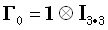(10)

where 1 is an M • 1 vector of ones. The null-eigenvectors correspond to the "spatial DC".

Because B is singular, the solution given by equation (2) is not valid. The general form of solution for this type of problem can be found in lemma 6.1 in . However, an equivalent simpler derivation will be developed here.

The new problem, which will now be reformulated, can be solved in two steps. In the first step, the problem to be solve is: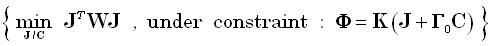(11)

Note that the minimization is with respect to J, for some given C, where C is a 3·1 vector of unknown coefficients, expressing the contribution of the DC level current density. Note that, by definition 0 ≡ 0 .

The solution to the problem expressed in equation (11) is: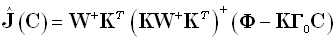(12)

which depends on C.

The second step consists of solving the problem: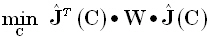(13)

which is equivalent to: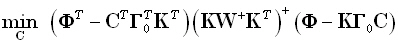(14)

The solution is: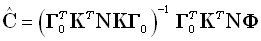(15)

with: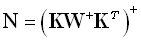(16)

Substituting 15 in 12 gives: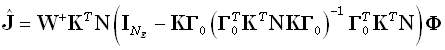(17)

Note that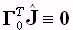.

The total current density estimator is: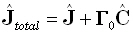(18)

or equivalently: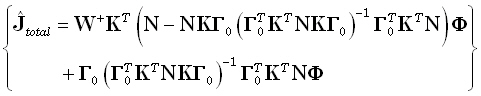(19)

An important comment:

This implements LORETA, without weights, and with boundary condition different from that used previously.

______________________________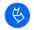Official journal of the International Society for Bioelectromagnetism